# Comparison of means: the t test

A t test is used to compare the means of two data sets, and it relies on calculation of a test statistic called t. This statistic is derived from the two data sets and it is defined as the difference between the means of the two data sets, x̅1 and x̅2 (or the difference between a mean x̅ and a reference value, xref) divided by the pooled standard error of the mean(s).

The standard error is a measure of the unreliability of a statistic. The standard error of a mean is:$SE_x = \sqrt{ \frac{s^2}{n} }$

The unreliability of an estimate of the population mean increases with the variance of the sample you are using to estimate it (hence the variance, s2, in the numerator) but decreases with sample size (hence n in the denominator).

The t statistic is calculated as follows:$t = \frac{ | \bar{x}_1 - \bar{x}_2 | }{ \sqrt{ \frac{ (n_1 - 1)s_1^2 + (n_2 - 1)s_2^2 }{ n_1 + n_2 - 2 } \cdot ( \frac{1}{n_1} + \frac{1}{n_2} ) } }$

Where x̅, s and n are the mean, standard deviation and sample size of the two data sets.

A t statistic can also be used to calculated from the mean of a single data set and a reference value, xref:$t = \frac{ | \bar{x} - x_{ref} | }{ \sqrt{ \frac{s^2}{n} } }$

The reference distribution for the t test is Student’s t. Like Fisher’s F, this reference distribution has two parameters: the number of degrees of freedom remaining in the first data set, and the number of degrees of freedom remaining in the second data set. As you are comparing one mean from each data set, then the remaining degrees of freedom for each data set is n−1, and the total number of degrees of freedom is n1+n2−2.

A t test compares the t statistic from your empirical data with the Student’s t value you’d expect under the null hypothesis, given the degrees of freedom in the two data sets. The p value of the test is the probability of obtaining a test t statistic at least as extreme as the one that was actually observed, assuming that the null hypothesis (“the means are equal”) is true. i.e. The p value is the probability of observing your data (or something more extreme), if the means really are equal.

The comparison is only valid if the sample data sets are:

• Representative of the larger population.
• Normally distributed. If the normality assumption is badly violated, a non-parametric alternative such as Wilcoxon rank sum (“Mann-Whitney U“) test, using wilcox.test(), might be an appropriate alternative.
• Independent of one another. A modification of the t test can be made for certain kinds of dependent data (e.g. where the samples in each group fall into natural pairs).
• Homoscedastic; that is they have the same variance. You can test for this assumption using the F test. If the two samples have difference variances, they are heteroscedastic. The Welch modification of Student’s t test can be used if this assumption is broken.

Do not use a Student’s t test if these assumptions are broken.

In R, a t-test is performed using t.test(). Here we generate thirty items of random normal data using rnorm() and compare two such data sets. We add 10 to all the data in dataset2 to make the means differ. The output of your code will differ as the data is random (if you’re very un/lucky, the difference may not even be significant!):

dataset1 <- rnorm(30)
dataset2 <- 10 + rnorm(30)
t.test( dataset1, dataset2 )
        Welch Two Sample t-test
data: dataset1 and dataset2
t = -46.0075, df = 56.604, p-value < 2.2e-16
alternative hypothesis: true difference in means is not equal to 0
95 percent confidence interval:
-10.444369  -9.572988
sample estimates:
mean of x   mean of y
0.01425418   10.02293268

Here the p value is less than 0.05, so we can say the difference in means is significant.

In R, the default t.test() actually assumes the variances are unequal (heteroscedastic), as this is very common, and it automatically uses Welch’s modification for unequal variances, which is actually rather less gnarly to look at in equation form:$t = \frac{ | \bar{x}_1 - \bar{x}_2 | }{ \sqrt{ \frac{s_1^2}{n_1} + \frac{s_2^2}{n_2} } }$

The degrees of freedom for Welch’s modification are no longer an integer, but instead:$df = \frac{ ( s_1^2/n_1 + s_2^2/n_2 )^2 }{ \frac{(s_1^2 / n_1)^2}{ n_1 - 1} + \frac{ (s_2^2 / n_2)^2 }{ n_2 - 1} }$

You can force R to use Student’s original t test for homoscedastic data by setting the option var.equal=TRUE.

# The code below should only be used if an F test indicates the variances of
# the two data sets are equal

t.test( dataset1, dataset2, var.equal=TRUE )

# The code below has the same effect as calling
t.test( dataset1, dateset2 )

# i.e. it uses Welch's modification to account for the difference in variances
t.test( dataset1, dataset2, var.equal=FALSE)

In general, you should use an F test to see whether the variances of your two data sets are equal: if so, use Student’s t (var.equal=TRUE); if not, use Welch’s modification of t, (var.equal=FALSE).

As with plot(), it is usually more convenient to use the ‘modelled-by’ tilde ~ operator to perform t tests within named data frames, rather than supplying the test with two vectors of data.

Here we t test the dog-whelk data in dog_whelks.csv that you already made in the exercises of an earlier post. As we have already established that the variances are not significantly different, we should use Student’s t rather than the Welch modification:

dog.whelks<-read.csv( "H:/R/dog_whelks.csv" )
t.test( Height ~ Exposure, data = dog.whelks,
var.equal=TRUE )
        Two Sample t-test
data:  Height by Exposure
t = -8.6399, df = 54, p-value = 9.274e-12
alternative hypothesis: true difference in means is not equal to 0
95 percent confidence interval:
-9.989079   -6.226306
sample estimates:
mean in group Exposed   mean in group Sheltered
20.19231                28.30000

Do a t test on the data in the dog.whelks data frame, using Height as the dependent variable, and grouping the data into two sets according to the Exposure factor

You should never put raw R output into written prose. If you wish to include the results of the F and t tests on the dog-whelk data above in a report, they should be written long-hand. In typical scientific literature, this might be something like…

Dog-whelk shell-height on exposed beaches was not significantly more variable than on sheltered beaches (F=1.77, p=0.13), based on a sample size of 25. However the shells on sheltered beaches were found to be significantly taller, by 10 mm (t=6.27, p ≪0.001).

…although this style of report mixes up Fisher and Neyman-Pearson approaches to hypothesis testing: by reporting a p value, you’re probably trying to persuade people of the importance of the ‘highly significant’ t test result (Fisher); however you’re also reporting your acceptance of the alternative hypothesis (Neyman-Pearson) under which the p value is irrelevant (except insofar as it is smaller than 0.05), but the power (which you’ve not stated, let alone used to determine an appropriate sample size!) is critical to the interpretation of the results. Ho hum. To do Neyman-Pearson properly, you should do a power calculation, for which you will find the function power.t.test useful.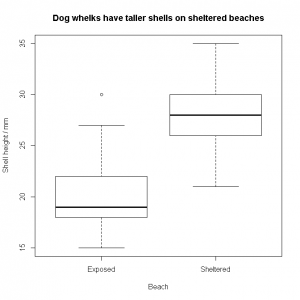You can select the heights of the sheltered-beach shells by using tapply(). This applies a function to a vector of data, grouped by another vector of the same length containing the levels of a factor.

N.B. The “t” in tapply() has nothing to do with the “t” in t– test: there are several other apply() functions with similarly cryptic names.

Remembering that the dog.whelks data frame looks like this:

   Height  Exposure
1      25  Sheltered
2      30  Sheltered
3      29  Sheltered
…
54     20   Exposed
55     24   Exposed
56     30   Exposed

We can apply the mean() function to the Height column (dog.whelks$Height) grouped by the Exposure factor (dog.whelks$Exposure) using this syntax:

# tapply( vector.to.group, factors.to.group.by, function.to.call )
means<-tapply( dog.whelks$Height, dog.whelks$Exposure, mean )
means["Sheltered"]-means["Exposed"]
Sheltered
8.107692

Shells from sheltered beaches are 8 mm taller. There are several simpler ways of working this out, e.g. using the linear model function lm(), which we will cover in later posts.

lm( Height ~ Exposure, data = dog.whelks )

To check the normality assumption for both F and t tests holds, you can use a qqnorm() plot and/or a hist() plot.

The normal quantile-quantile (QQ) plot plots the real data against theoretical normally-distributed data of the same mean and standard error. If the real data are normally distributed, then the QQ plot should be a straight line. Curved or S-shaped QQ plots are indicative of non-normal data. Note the use of the [ condition ] indexing brackets to extract out from the vector only those items for which the Exposure factor is “Sheltered“: the overall data set will certainly not be normal, as the t-test shows the two exposures come from two different (normal) distributions:

qqnorm( dog.whelks$Height[ dog.whelks$Exposure=="Sheltered" ] )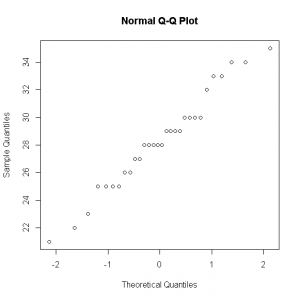A histogram of the data should look like a normal bell-curve, without obvious tails (skew) or peakiness/flatness (kurtosis); however, for small data sets, the histogram is unlikely to be smooth and ‘perfect’ looking, as you can see below. For this reason, the normal QQ plot is generally the preferred way of seeing whether data are normal enough to be analysed using F and t tests.

hist( dog.whelks$Height[dog.whelks$Exposure=="Sheltered"] )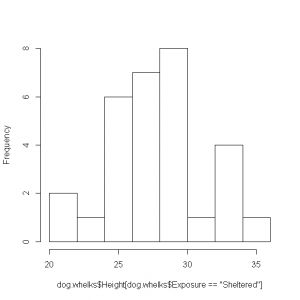You might like to plot a QQ plot and a histogram of the “Exposed” portion of the data set, and perhaps look at a histogram of the entire data set, to confirm that it is indeed composed of two overlapping (approximately) normal curves.

Some things to bear in mind when writing summaries of statistics in prose:

• Do not include raw R output.
• Include the name of the test you performed, the test statistic and its p value.
• R will usually give you the p value itself, or – as here – an indication that it is lower than the minimum value it can calculate. There is no need to additionally look up your t statistic (6.27) in tables to confirm that this is indeed associated with a p value below the critical value of your choice. This makes about as much sense as performing the calculation 34378 times 83623 on a calculator, ignoring the result, and then using a slide-rule to estimate the product instead.
• Don’t simply say a difference in means investigated by a t test is “significant”. Say which level of the factor is associated with the larger or smaller mean: it’s not just that the dog-whelk heights were different, the dog-whelks on sheltered beaches were – on average – taller. This is acceptable, as a one-tailed t test (which would test for ‘taller’ or ‘shorter’) will always have a p value less than 0.05 if the corresponding two-tailed t test  has a p value less than 0.05.
• More generally, make sure the reader knows why you’ve done the statistics: it’s too easy to forget that the whole point is to see whether some dependent variable of interest (dog-whelk shell height) can be modelled/explained using an independent variable of interest (type of beach the shell is found on).
• Give an indication of the sample size.

# Exercises

Use F and t tests to investigate the following data sets. For each data set, write a short piece of prose describing the results of the statistical analysis. Plot suitable graphs for each set to accompany the text.

1. The data in pollen_tubes.csv show the growth rate of pollen tubes from Impatiens pollen, grown in an agar-solidified basal medium containing sucrose, vs. the same medium with borate and calcium supplementation.
2. The data in pipette_check.csv show the mass (g) of water measured when two brands of 1 mL adjustable pipettes are set to their maximum volume and used to pipette pure water. Which brand of pipette is better?
3. You intend collecting some data on the growth rate of bacteria in a liquid broth culture. Due to space limitations, you can only use 8 flasks. You intend on running 4 flasks as controls, and spiking another 4 with silver nitrate to see what effect it has on the exponential growth rate. Some preliminary experiments indicate that the control growth rate is typically 0.02 min−1 with a standard deviation of 0.005 min−1. Assuming the standard deviation will be the same in the silver-spiked flasks, what is the minimum difference in mean growth rate between the control and silver-spiked flasks that you can expect to reliably detect (α=0.05, β = 0.20)? You will probably want to use power.t.test rather than trying to simulate the data.

1. The rate of growth of germinating pollen tubes (n=15 per treatment) in supplemented medium was found to be significantly larger (t=3.73, p=0.00085). The growth rate (mean±sd / µm hr−1) was increased from 560(±224) in the basal medium to 891(±261), but no effect was observed on the variance (F=0.73, p=0.56).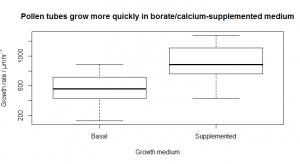pollen.tubes<-read.csv( "H:/R/pollen_tubes.csv" )
var.test( Growth.Rate ~ Medium, data = pollen.tubes )
        F test to compare two variances
data:  Growth.Rate by Medium
F = 0.7309, num df = 14, denom df = 14, p-value = 0.5653
alternative hypothesis: true ratio of variances is not equal to 1
95 percent confidence interval:
0.2453878 2.1770768
sample estimates: ratio of variances
0.7309091
t.test( Growth.Rate ~ Medium, data = pollen.tubes, var.equal=TRUE )
       Two Sample t-test data:
Growth.Rate by Medium
t = -3.7345, df = 28, p-value = 0.0008522
alternative hypothesis: true difference in means is not equal to 0
95 percent confidence interval:
-514.1032 -149.8968
sample estimates:
mean in group Basal       mean in group Supplemented
559.3333                  891.3333
tapply( pollen.tubes$Growth.Rate, pollen.tubes$Medium, mean )
       Basal        Supplemented
559.3333     891.3333
tapply( pollen.tubes$Growth.Rate, pollen.tubes$Medium, sd )
       Basal        Supplemented
223.7389     261.7037
# Plot the data with suitable title
plot(
Growth.Rate ~ Medium,
data = pollen.tubes,
xlab = "Growth medium",
ylab = expression("Growth rate / µm " * hr^-1),
main= "Pollen tubes grow more quickly in borate/calcium-supplemented medium"
)
1. The Piprecision pipettes have a variance at least twice as high as that of the Accurette model (F=0.23, p=0.00016), indicating the Acurrette pipettes are more precise. No difference in accuracy was observed (t=0.48, p=0.063) across the two brands.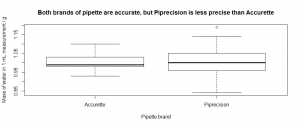pipette.check<-read.csv( "H:/R/pipette_check.csv" )

var.test( Mass ~ Brand, data=pipette.check )
        F test to compare two variances
data:  Mass by Brand
F = 0.2298, num df = 29, denom df = 29, p-value = 0.0001639
alternative hypothesis: true ratio of variances is not equal to 1
95 percent confidence interval:
0.1093666  0.4827644
sample estimates:
ratio of variances
0.2297789
t.test( Mass ~ Brand, data=pipette.check )
        Welch Two Sample t-test
data:  Mass by Brand
t = -0.4835, df = 41.659,
p-value = 0.6313
alternative hypothesis: true difference in means is not equal to 0
95 percent confidence interval:
-0.03967369  0.02434036
sample estimates:
mean in group Accurette  mean in group Piprecision
0.999000                   1.006667
tapply( pipette.check$Mass, pipette.check$Brand, mean )
  Accurette  Piprecision
0.999000     1.006667
tapply( pipette.check$Mass, pipette.check$Brand, sd )
  Accurette  Piprecision
0.03754078  0.07831560
# Plot the data with suitable title
plot(
Mass ~ Brand,
data = pipette.check,
xlab = "Pipette brand",
ylab = "Mass of water in 1 mL measurement / g",
main="Both brands of pipette are accurate, but Piprecision is less precise than Accurette"
)
1. power.t.test takes exactly four of a sample size per group (n), a difference in means (delta), a power (power = 1 − β), a standard deviation (sd), and a significance level (sig.level = α) and returns the fifth parameter. We want the difference in means here, which turns out to be 0.0119, which is a very substantial fraction of the growth rate in the control (0.02), i.e. we would only be able to reliably detect a difference in growth rate if the silver causes the growth rate constant to (slightly more than) halve in value. This is why doing power calculations is worthwhile!
power.t.test( n=4, power=0.8, sd=0.005, sig.level=0.05 )
Two-sample t test power calculation
n = 4
delta = 0.01190707
sd = 0.005
sig.level = 0.05
power = 0.8
alternative = two.sided
NOTE: n is number in *each* group

Next up… Linear regression.

This site uses Akismet to reduce spam. Learn how your comment data is processed.